Miscellaneous

Chapter 10 Class 11 Straight Lines
Serial order wise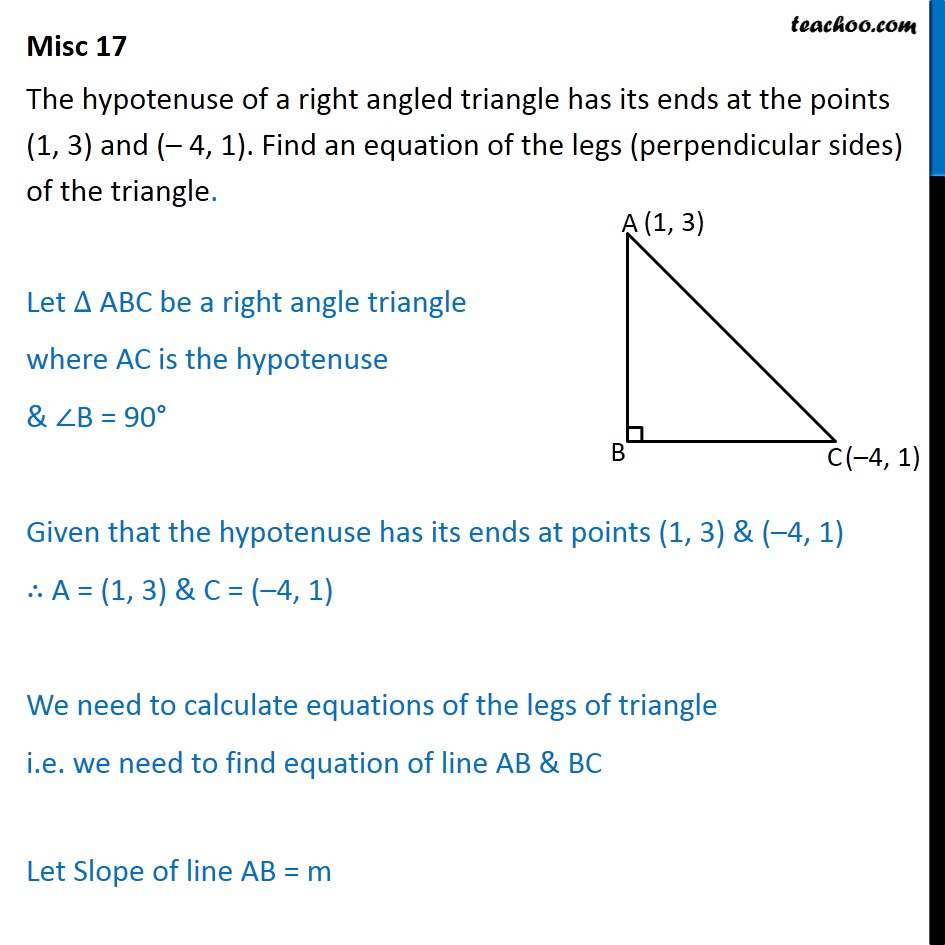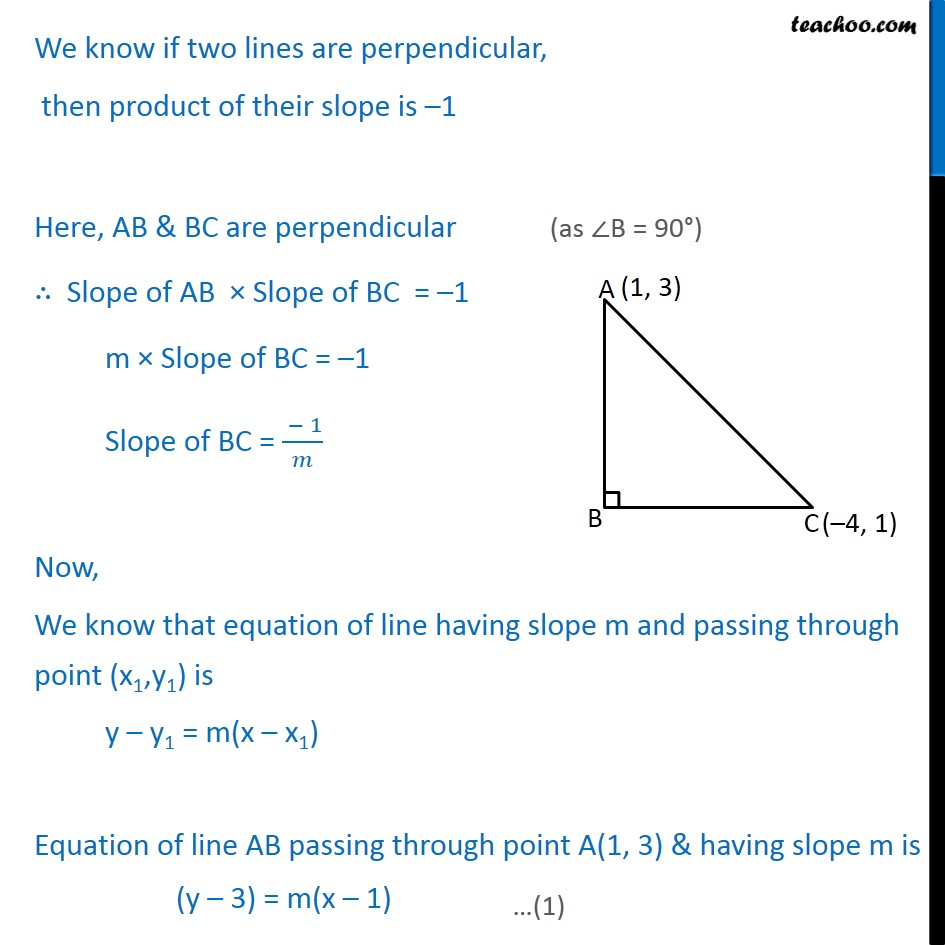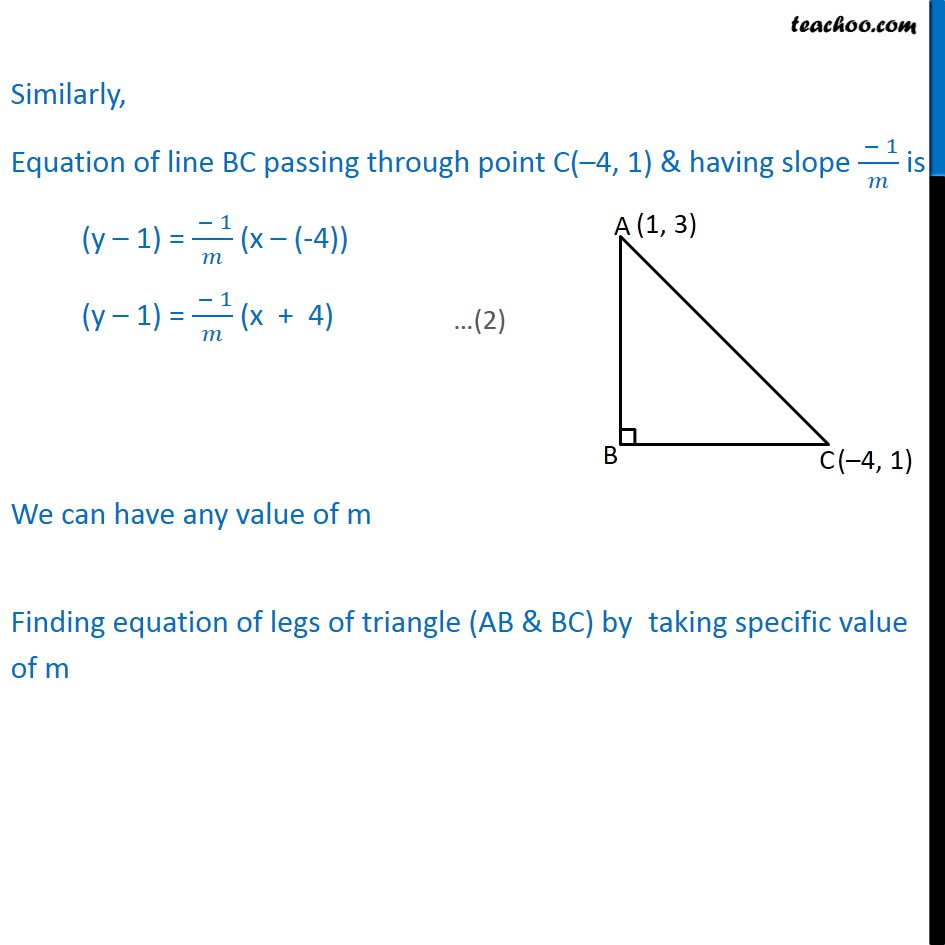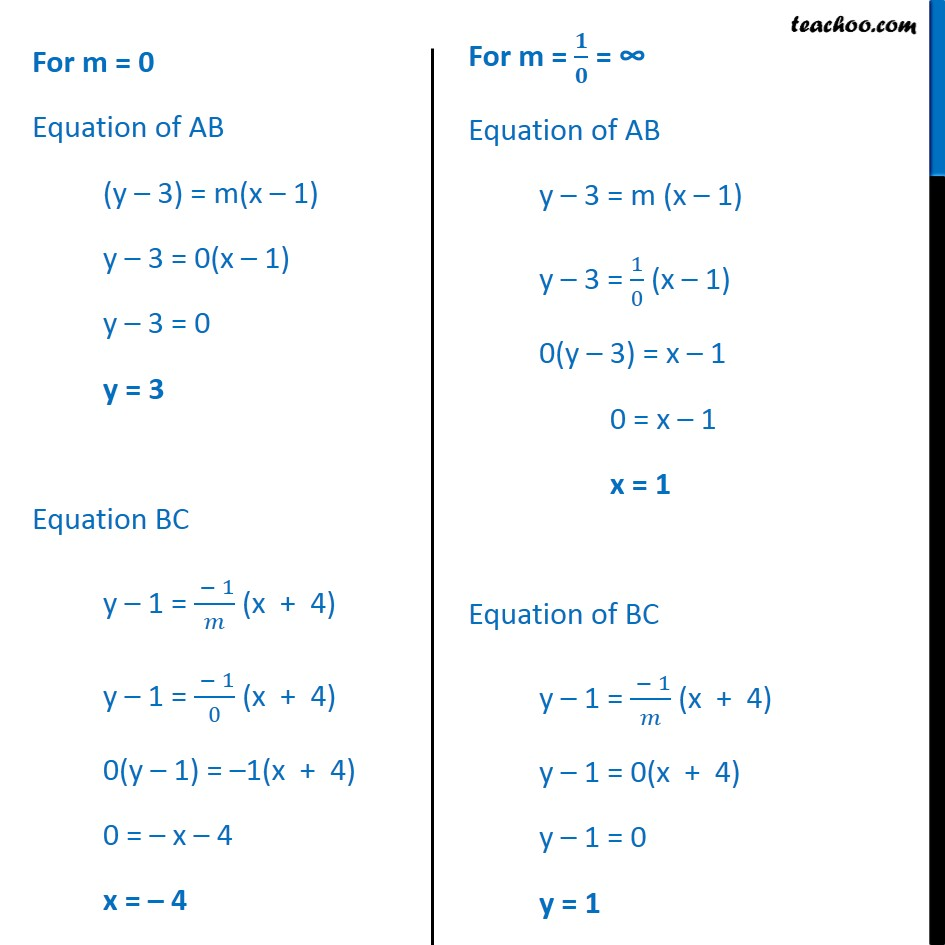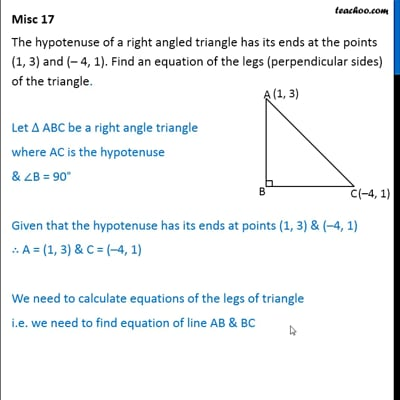This video is only available for Teachoo black users

Get live Maths 1-on-1 Classs - Class 6 to 12

### Transcript

Misc 17 The hypotenuse of a right angled triangle has its ends at the points (1, 3) and ( 4, 1). Find an equation of the legs (perpendicular sides) of the triangle. Let ABC be a right angle triangle where AC is the hypotenuse & B = 90 Given that the hypotenuse has its ends at points (1, 3) & ( 4, 1) A = (1, 3) & C = ( 4, 1) We need to calculate equations of the legs of triangle i.e. we need to find equation of line AB & BC Let Slope of line AB = m We know if two lines are perpendicular, then product of their slope is 1 Here, AB & BC are perpendicular Slope of AB Slope of BC = 1 m Slope of BC = 1 Slope of BC = ( 1)/ Now, We know that equation of line having slope m and passing through point (x1,y1) is y y1 = m(x x1) Equation of line AB passing through point A(1, 3) & having slope m is (y 3) = m(x 1) Similarly, Equation of line BC passing through point C( 4, 1) & having slope ( 1)/ is (y 1) = ( 1)/ (x (-4)) (y 1) = ( 1)/ (x + 4) We can have any value of m Finding equation of legs of triangle (AB & BC) by taking specific value of m For m = 0 Equation of AB (y 3) = m(x 1) y 3 = 0(x 1) y 3 = 0 y = 3 Equation BC y 1 = ( 1)/ (x + 4) y 1 = ( 1)/0 (x + 4) 0(y 1) = 1(x + 4) 0 = x 4 x = 4